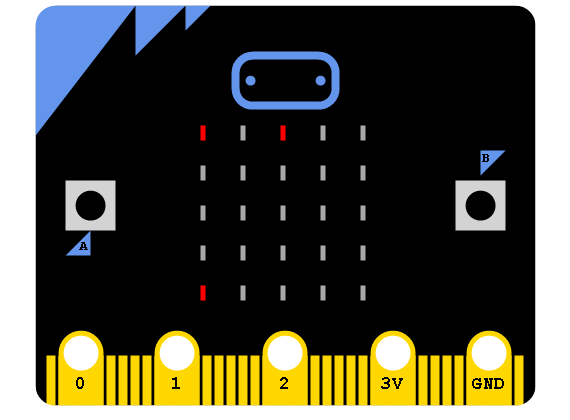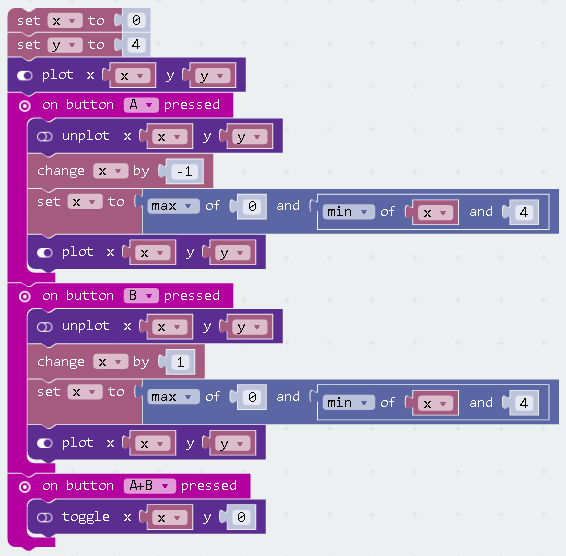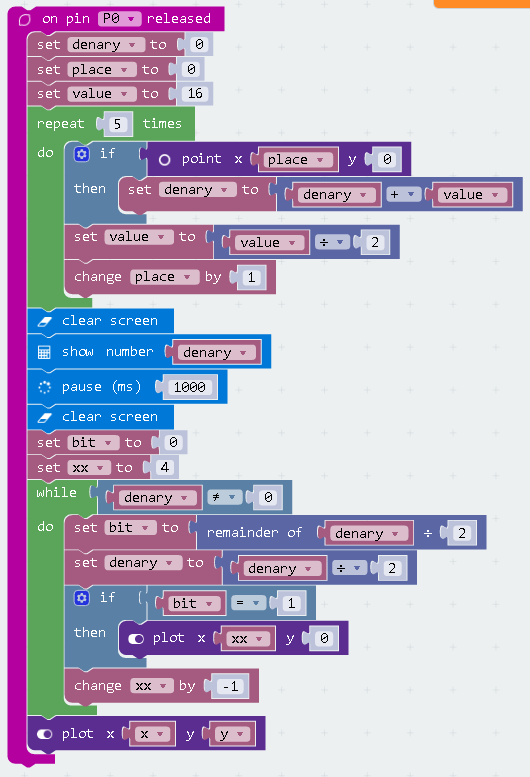# BBC micro:bit Binary Conversion

## Introduction

Using on/off LEDs as binary digits on the top row of the LED matrix, we can represent binary patterns for numbers from 0 to 31. This page shows a method of entering a number in this range, converting it into a denary number and then converting that number back to its original form. Do revise your binary before continuing, this page is about the program.

## Programming

The first thing to develop is a way of interacting with the micro:bit to input binary numbers into the micro:bit. The following display shows a micro:bit with the binary pattern for 20 entered. The dot in the bottom row is moved to the left and right using the A and B buttons. Pressing the two buttons together will toggle the top row pixel in the same column as the pointer.This the basic code for this part of the project.Notice that we are plotting and unplotting the pixel rather than using a sprite. This allows us better control of the screen than if we start monkeying with the game library.

There is a nice little technique used to keep the pointer pixel on the screen,You can use this technique any time you want to clamp or restrict a variable's value to a range of integers. The 0 and 4 in this statement represent the smallest and largest allowable values.

Now for the hard work. To convert the binary number into denary, the user will need to touch pin 0 annd GND at the same time, when they let go, the denary number is displayed on the screen. After a brief pause, the binary pattern is shown again and the user can change the number or look at the denary again.

If we stick to using the blocks, we don't have access to any easy way to store binary patterns. So, the binary pattern is converted into a denary number that we can store in a variable. In order to return the user to the pattern after we've cleared the screen, we're going to have to convert it back to binary. This is a task we can do joyfully.The blue blocks in the middle of this event are the ones that show the denary number. Everything before them is about converting binary to denary. Everything after the middle blue blocks is about converting denary to binary.

### Converting To Denary

The method used to convert to denary is easy to understand if you make a table and trace what happens to the values stored in the variables over each of the 5 repetitions. The following image shows a binary pattern that a user could enter using the method we have developed,If we trace the way that the values stored in our variables change, we can see how this works,

RepetitionPoint (place,0)denaryvalueplace
- 0
-  160
1true
1 16
1  81
2false
2 16
2  42
3true
3 20
3  23
4false
4 20
4  14
5true
5 21
5  05

Notice that the last 2 assignments aren't used. A better algorithm would not need that to happen.

### Converting To Binary

This is the standard algorithm for converting a denary number to binary. This implementation relies and the fact that decimal numbers are not supported in the block editor to round down any divisions that result in a decimal. The equivalent table to create the binary pattern for the number 21 is as follows,

denarybitxxdenary == 0
2104
true
1
10
3
true
0
5
2
true
1
2
1
true
0
1
0
true
1
0
-1
false

The first line of the table shows the values the variables have at the start. The next 5 values of the variable bit are used to determine whether or not the pixel in column xx should be lit. A 1 means it's lit.

### JavaScript

It loooks a little more compact in the JavaScript.

```let xx = 0
let bit = 0
let value = 0
let place = 0
let denary = 0
let x = 0
let y = 4
led.plot(x, y)
input.onButtonPressed(Button.A, () => {
led.unplot(x, y)
x += -1
x = Math.max(0, Math.min(x, 4))
led.plot(x, y)
})
input.onButtonPressed(Button.B, () => {
led.unplot(x, y)
x += 1
x = Math.max(0, Math.min(x, 4))
led.plot(x, y)
})
input.onButtonPressed(Button.AB, () => {
led.toggle(x, 0)
})
input.onPinReleased(TouchPin.P0, () => {
denary = 0
place = 0
value = 16
for (let i = 0; i < 5; i++) {
if (led.point(place, 0)) {
denary = denary + value
}
value = value / 2
place += 1
}
basic.clearScreen()
basic.showNumber(denary)
basic.pause(1000)
basic.clearScreen()
bit = 0
xx = 4
while (denary != 0) {
bit = denary % 2
denary = denary / 2
if (bit == 1) {
led.plot(xx, 0)
}
xx += -1
}
led.plot(x, y)
})

```

## Challenges

1. You could use the first 4 pixels of the first two rows of the matrix to make up the 8 bits of a byte. You could use a tilting gesture to move the pointer up and down a row. This would allow you to enter numbers from 0 to 255.
2. You have the basis for a quiz here. You could generate the binary patterns or the denary numbers at random and have the player convert them to increase their score.